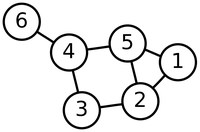# Glossary

An adjacency list is a two-column matrix that abstractly represents the edge relations of a graph without needing to physically draw the graph. Each row of the adjacency list encodes an edge $\{u, v\}$ by placing $u$ in the first column and $v$ in the second column (or vice-versa).

For example, consider the following graph:The adjacency list of this graph is given below (the rows could be given in any order, and the elements of any given row could be transposed).

1 2
1 5
2 3
2 5
3 4
4 5
4 6 

If a graph is instead directed, then to encode the directed edge $(u, v)$ in the adjacency list, $u$ must be placed in column 1 and $v$ in column 2. Consider the following directed graph.The following adjacency list for this graph correctly encodes the orientation of each edge; the edges may be given in any order, as long as the tail of each edge is provided in column 1.

3 8
3 10
5 11
7 8
7 11
8 9
11 2
11 9
11 10